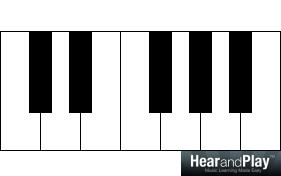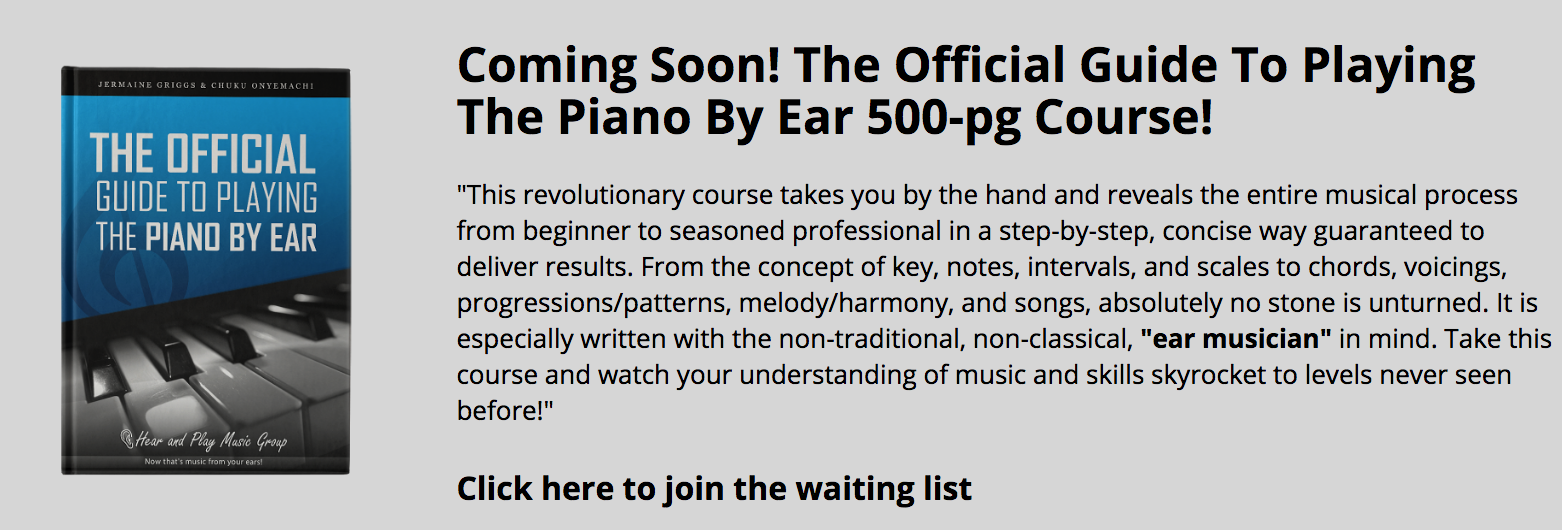• # Fullproof Formulas For Mastering Triads And Seventh Chords In Any KeySo you want to master triads and seventh chords in any key?

Well, there’s good news for you. It’s very possible to master four triad types and five seventh chord types using the formulas I’m about to show you in this lesson.

If you’re like me, there’s every tendency that you shy away from formulas due to the fact that you are don’t find mathematics interesting. But one thing I guarantee you is that these formulas are easy to learn and recall.

Alright! We’re good to go. Give me your undivided attention for the next 8 minutes or so.

## A Quick Review On Triads And Seventh Chords

When a collection of three or more related notes are played or heard together, a chord is formed. Let’s go ahead and look at two important classes of chords — triads and seventh chords.

A triad is a chord of three notes. A classic example of a triad is the C major triad:…which consists of C, E, and G — which are related by the C major scale:The relationship between the tones of the C major triad is that they are the first, third, and fifth tones of the C major scale.

The notes are also related by the distance between them. The interval between the successive notes of the C major triad are third intervals and this explains why C to E:…is a third interval (encompassing three scale tones of the C major scale: C, D, and E), and so is E to G:There are four main triad qualities:

“Here’s A Reference To These Triads In C…”### Seventh Chords — Explained

Seventh chords are named after their width. A seventh chord encompasses seven scale tones (which is an interval of a seventh. Using the C major triad (as a reference):…we can form a seventh chord by adding a B-note:…to produce the C major seventh chord:The C major seventh chord is considered to be a seventh chord because when played in root position, it encompasses a seventh. For example, the interval between the lowest-sounding note in the C major seventh chord (which is C) and the highest-sounding note (which is B):…is a seventh interval.

There are five commonly used seventh chord types:

The major seventh chord

The minor seventh chord

The dominant seventh chord

The half-diminished seventh chord

The diminished seventh chord

“Here’s A Reference To These Triads In C…”

The C major seventh chord:The C minor seventh chord:The C dominant seventh chord:The C half-diminished seventh chord:The C diminished seventh chord:Submission: The appropriate spelling of the C diminished seventh triad is “C-Eb-Gb-Bbb”. The use of A in the virtual keyboard image above is deliberate and that’s because my illustrator doesn’t spell double flats yet.

## The Concept Of Chord Formulas

There are so many ways to learn and master chords and one of the ways we’re considering in this lesson is the use of chord formulas.

“Can Chords Be Formularized?”

Using the major scale (that you’re familiar  with), the tones of a chord can be represented and formularized and this is derived by the relationship between the tones of the chord and the tones of the major scale.

For example, the C dominant seventh chord:…can be formularized and this is derived by the relationship between the tones of the C dominant seventh chord (which are C, E, G, and Bb):…and the tones of the C major scale (which are C, D, E, F,G, A, B, and C):“Check It Out…”

The first tone of the C dominant seventh chord is C:…and that’s the first tone of the C major scale. Therefore, C is represented as 1.

The third tone of the C dominant seventh chord is E:…and that’s the third tone of the C major scale. Therefore, E is represented as 3.

The fifth tone of the C dominant seventh chord is G:…and that’s the fifth tone of the C major scale. Therefore, G is represented as 5.

The seventh tone of the C dominant seventh chord is Bb:…and that’s lower than the seventh tone of the C major scale (which is B) by a half-step. Therefore, Bb is represented as b7.

Altogether, the C dominant seventh chord can be formularized thus:

1   –   3   –   5   –   b7

…and this is in relationship with the C major scale.

So, using any major scale, a corresponding dominant seventh chord can be derived using the “1-3-5-b7” chord formula.

Let’s go ahead and explore chord formulas for triads and seventh chords.

A triad is a chord of three notes and consists of the following components:

The root

The third

The fifth

Considering the tones of the four known triad types and the tones of the major scale will give us the chord formula for every triad type.

### Chord Formula For The Major Triad

The tones of the C major triad:..can be broken down thus:

The first tone of the C major triad is C:…and that’s the first tone of the C major scale. Therefore, C is represented as 1.

The third tone of the C major triad is E:…and that’s the third tone of the C major scale. Therefore, E is represented as 3.

The fifth tone of the C major triad is G:…and that’s the fifth tone of the C major scale. Therefore, G is represented as 5.

Altogether, the chord formula of the major triad is “1-3-5” and using this chord formula, the major triad can be derived from any major scale.

### Chord Formula For The Minor Triad

The tones of the C minor triad:..can be broken down thus:

The first tone of the C minor triad is C:…and that’s the first tone of the C major scale. Therefore, C is represented as 1.

The third tone of the C minor triad is Eb:…and that’s lower than the third tone of the C major scale (which is E) by a half-step. Therefore, Eb is represented as b3.

The fifth tone of the C major triad is G:…and that’s the fifth tone of the C major scale. Therefore, G is represented as 5.

Altogether, the chord formula of the major triad is “1-b3-5” and using this chord formula, the minor triad can be derived from any major scale.

Using the “1-b3-5” formula on the D major scale:…produces the following tones:

D which is the 1

F which is the b3 (of course the 3 is F#)

A which is the 5

So, the D minor triad (D, F, and A):…consists of the 1, b3, and 5 tones of the D major scale.

### Chord Formula For The Augmented Triad

The tones of the C augmented triad:..can be broken down thus:

The first tone of the C augmented triad is C:…and that’s the first tone of the C major scale. Therefore, C is represented as 1.

The third tone of the C augmented triad is E:…and that’s the third tone of the C major scale. Therefore, E is represented as 3.

The fifth tone of the C augmented triad is G#:…and that’s higher than the fifth tone of the C major scale (which is G) by a half-step. Therefore, G# is represented as #5.

Altogether, the chord formula of the augmented triad is “1-3-#5” and using this chord formula, the augmented triad can be derived from any major scale.

### Chord Formula For The Diminished Triad

The tones of the C diminished triad:..can be broken down thus:

The first tone of the C diminished triad is C:…and that’s the first tone of the C major scale. Therefore, C is represented as 1.

The third tone of the C diminished triad is Eb:…and that’s lower than the third tone of the C major scale (which is E) by a half-step. Therefore, Eb is represented as b3.

The fifth tone of the C diminished triad is Gb:…and that’s the fifth tone of the C major scale. Therefore, Gb is represented as 5.

Altogether, the chord formula of the major triad is “1-b3-b5” and using this chord formula, the diminished triad can be derived from any major scale.

## Chord Formulas For Seventh Chords

Content will be available soon.

## Final Words

Using the chord formulas covered in this lesson, any triad or seventh chord can be formed on the keyboard. However, you must be familiar with the major scale in all the keys before you can take advantage of these chord formulas.

In a subsequent lesson. we’ll learn how extended chords can be formularized using the concept of voicing formulas.

See you then!

The following two tabs change content below.#### Chuku Onyemachi

Head of Education at HearandPlay Music Group
Hello, I'm Chuku Onyemachi (aka - "Dr. Pokey") - a musicologist, pianist, author, clinician and Nigerian. Inspired by my role model Jermaine Griggs, I started teaching musicians in my neighborhood in April 2005. Today, I'm privileged to work as the head of education, music consultant, and chief content creator with HearandPlay Music Group sharing my wealth of knowledge with hundreds of thousands of musicians across the world.#### Latest posts by Chuku Onyemachi (see all)